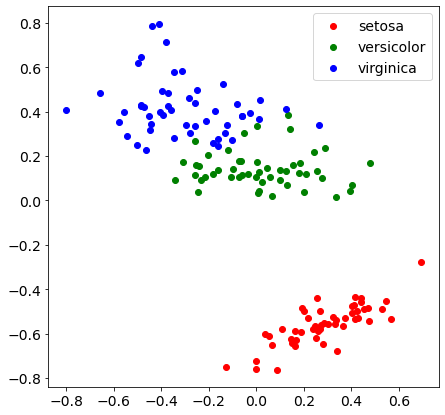# Visualize multidimensional datasets with MDS

Original article was published on Artificial Intelligence on MediumAs long as we work with two-dimensional datasets, a simple scatterplot can be quite useful to visualize patterns and events. If we work with three-dimensional data there’s still some chance to visualize something using 3d plots.

But what happens if we want to visualize higher-dimensional datasets? Things can become more difficult. Think about clustering problems. It would be very wonderful if we could visualize data in many dimensions in order to check whether there are some patterns or not.

Of course, we don’t have a multidimensional vision, so we must transform multidimensional data into 2d data. An algorithm able to do it is MDS.

# What is MDS?

MDS (multidimensional scaling) is an algorithm that transforms a dataset into another dataset, usually with lower dimensions, keeping the same euclidean distances between the points.

Keeping the distances is a very useful feature of MDS because it allows us to reasonably preserve patterns and clusters if, for example, we want to perform K-Means or other types of clustering.

So, for example, if we have a 4-dimensional dataset and want to visualize it, we can use MDS to scale it in 2 dimensions. The distances between points are kept as in the original dataset, so if data self-organizes in clusters, they can be visible even after the scaling procedure.

Of course, the coordinates of the new points in the lower dimension no longer have business value and are dimensionless. Value is carried by the shape of the scatterplot and by the relative distances between points.

It’s worth mentioning that a dataset should be normalized or standardized before giving it to MDS. That’s very similar to what we do with K-Means clustering, for example. The reason is very simple: we don’t want to give more weight to some features only because their order of magnitude is higher than others’. A simple 0–1 normalization will solve this problem effectively.

In Python, there’s a nice implementation in MDS under the module `manifold` of the package `sklearn`. Let’s see an example using the famous Iris dataset.

# An example in Python

We’re going to visualize the 4 features of the Iris dataset using MDS to scale them in 2 dimensions. First, we’ll perform a 0–1 scaling of the features, then we’ll perform MDS in 2 dimensions and plot the new data, giving each point a different color according to the target variable of the Iris dataset.

Let’s start importing some libraries.

`import numpy as npfrom sklearn.datasets import load_irisimport matplotlib.pyplot as pltfrom sklearn.manifold import MDSfrom sklearn.preprocessing import MinMaxScaler`

Now, let’s load the Iris dataset.

`data = load_iris()X = data.data`

We can now perform a 0–1 scaling with `MinMaxScaler`

`scaler = MinMaxScaler()X_scaled = scaler.fit_transform(X)`

Then, we apply the MDS procedure to get a 2-dimensional dataset. The random_state is set in order to make every plot reproducible.

`mds = MDS(2,random_state=0)X_2d = mds.fit_transform(X_scaled)`

Finally, we can plot the new dataset.

`colors = ['red','green','blue']plt.rcParams['figure.figsize'] = [7, 7]plt.rc('font', size=14)for i in np.unique(data.target): subset = X_2d[data.target == i] x = [row for row in subset] y = [row for row in subset]plt.scatter(x,y,c=colors[i],label=data.target_names[i])plt.legend()plt.show()`

And here’s the result.